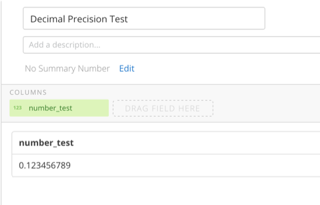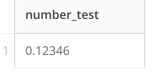# Domo Rounds Decimal Precision when using Nested Dataflows

I am building out financial calculations with Foreign Exchange Rates in a dataflow. These rates require accurate precision because they are used as multipliers against billions of dollars, so rounding the precision causes variances in the tens of thousands of dollars.

Scenario: Domo is rounding the precision of numbers to 5 decimal places when using the Dataflow as Input in another Dataflow.

Here is how to replicate the issue:

Make a sample dataflow with one transform to load a number with precision > 5 as in:

`SELECT 0.123456789 as number_test`

Good: Data is properly stored as seen by making a sample card from the dataset.Bad: If you use the dataflow as input in another dataflow, numbers are automatically rounded to 5 places. This makes the number inaccurate for calculations in the second dataflow.I have tried casting the precision when creating the data, but storage isn’t the problem. Also tried casting the precision in the second dataflow, but it's already rounded when the dataset loads. Something about how Domo loads the output of the dataflow is causing the rounding.

Is this issue known and does anyone know of a way to retain the full decimal precision of a dataflow output?

Jacob Folsom
**Say “Thanks” by clicking the “heart” in the post that helped you.
**Please mark the post that solves your problem by clicking on "Accept as Solution"